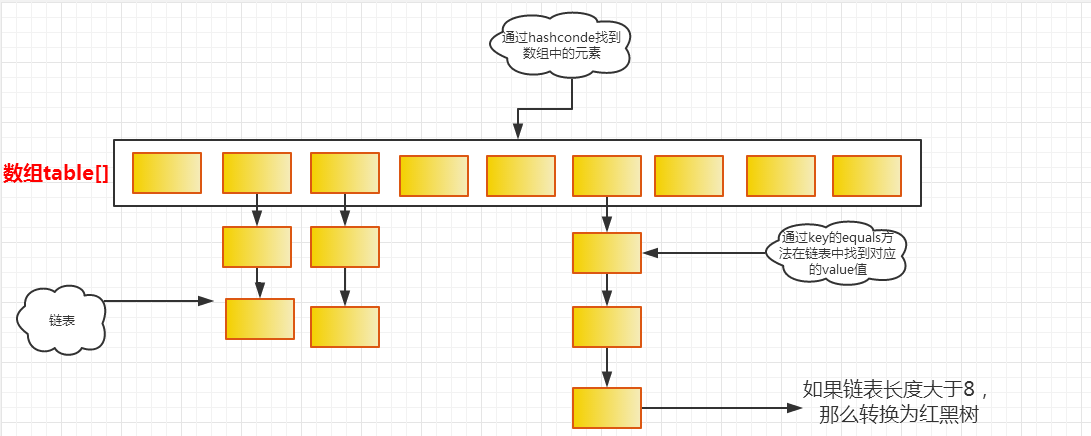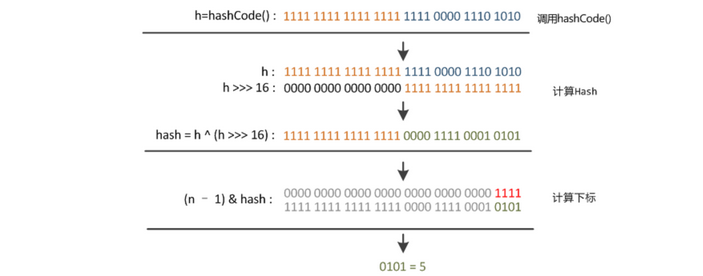# HashMap详解

http://www.cnblogs.com/DSNFZ/articles/7634042.html

# 1）HashMap概述

HashMap是基于哈希表的map接口的非同步实现，此实现提供所有可选的映射操作，并允许使用null值和null键。此类不保证映射的顺序，特别是它不保证该顺序恒久不变。

# 2）HashMap数据结构java中采用的便是链地址法，便是每个数组元素上都是一个链表。当数据被hash后，得到数组下标，将数据放在对应数组下标的链表上

 static class Node<K,V> implements Map.Entry<K,V> {
final int hash;
final K key;
V value;
Node<K,V> next;

Node(int hash, K key, V value, Node<K,V> next) {
this.hash = hash;
this.key = key;
this.value = value;
this.next = next;
}
}

node是hashmap的一个内部类，用来储存数据和保持链表结构的。它的本质就是一个映射（键值对）

hashmap中又一个很重要的字段就是Node[] table。如上图所示，这就是hashmap的基本结构，构成链表的数组。


//默认的初始容量
static final int DEFAULT_INITIAL_CAPACITY = 1 << 4; // aka 16
//最大容量2^30
static final int MAXIMUM_CAPACITY = 1 << 30;
//加载因子(如果值太高, 虽说会提高空间利用率, 但是加大查找的开销)
static final float DEFAULT_LOAD_FACTOR = 0.75f;
//由链表转换成树的阈值
static final int TREEIFY_THRESHOLD = 8;
//由树转换成链表的阈值
static final int UNTREEIFY_THRESHOLD = 6;
//当桶中的bin被树化时最小的hash表容量。（如果表中的节点太多，则重新调整表大小）这个值至少是TREEIFY_THRESHOLD的4倍。
static final int MIN_TREEIFY_CAPACITY = 64;
//hashmap结构修改的次数(注意是结构)
transient int modCount;
// 下次扩容阀值(容量*加载因子)
int threshold;
//映射对的数量
transient int size;

上面这些参数的是非常非常重要的，其重要性相当于hashmap的数据结构的重要性。在本篇中，我们运用到并重点讲解的为一下几个参数：


static final int DEFAULT_INITIAL_CAPACITY = 1 << 4;
static final float DEFAULT_LOAD_FACTOR = 0.75f;
transient int size;
transient int modCount;
int threshold;
final float loadFactor;

size就是在这个hashmpa中实际存在的node数量。modCount便是hashmap结构修改的次数。在之前对iterator（迭代器）进行讲解的时候我已经进行了说明，需要注意的是在hashmap中modcount指的是结构更改的次数，例如添加新的node，但是如果是替换原有node的value，modcount是不变的，因为它不属于结构变化。

Hashtable初始化桶大小为11，就是桶大小设计为素数的应用（Hashtable扩容后不能保证还是素数）。HashMap采用这种非常规设计，主要是为了在取模和扩容时做优化，同时为了减少冲突，HashMap定位哈希桶索引位置时，也加入了高位参与运算的过程。

# 3）确认hashmap索引位置

    static final int hash(Object key) {
int h;
return (key == null) ? 0 : (h = key.hashCode()) ^ (h >>> 16);
}# 4）hashmap的put实现方法：

put函数大致的思路为：

1. 对key的hashCode()做hash，然后再计算index;
2. 如果没碰撞直接放到bucket里；
3. 如果碰撞了，以链表的形式存在buckets后；
4. 如果碰撞导致链表过长(大于等于TREEIFY_THRESHOLD)，就把链表转换成红黑树；
5. 如果节点已经存在就替换old value(保证key的唯一性)

public V put(K key, V value) {
return putVal(hash(key), key, value, false, true);
}

final V putVal(int hash, K key, V value, boolean onlyIfAbsent, boolean evict) {
Node<K, V>[] tab;
Node<K, V> p;
int n, i;
if ((tab = table) == null || (n = tab.length) == 0)
n = (tab = resize()).length; // 当数组table为null时, 调用resize生成数组table, 并令tab指向数组table
if ((p = tab[i = (n - 1) & hash]) == null) // 如果新存放的hash值没有冲突
tab[i] = newNode(hash, key, value, null); // 则只需要生成新的Node节点并存放到table数组中即可
else { // 否则就是产生了hash冲突
Node<K, V> e;
K k;
if (p.hash == hash && ((k = p.key) == key || (key != null && key.equals(k))))
e = p; // 如果hash值相等且key值相等, 则令e指向冲突的头节点
else if (p instanceof TreeNode) // 如果头节点的key值与新插入的key值不等, 并且头结点是TreeNode类型,说明该hash值冲突是采用红黑树进行处理.
e = ((TreeNode<K, V>) p).putTreeVal(this, tab, hash, key, value); // 向红黑树中插入新的Node节点
else { // 否则就是采用链表处理hash值冲突
for (int binCount = 0;; ++binCount) { // 遍历冲突链表, binCount记录hash值冲突链表中节点个数
if ((e = p.next) == null) { // 当遍历到冲突链表的尾部时
p.next = newNode(hash, key, value, null); // 生成新节点添加到链表末尾
if (binCount >= TREEIFY_THRESHOLD - 1) // 如果binCount即冲突节点的个数大于等于 (TREEIFY_THRESHOLD(=8) - 1),便将冲突链表改为红黑树结构, 对冲突进行管理,
// 否则不需要改为红黑树结构
treeifyBin(tab, hash);
break;
}
if (e.hash == hash && ((k = e.key) == key || (key != null && key.equals(k)))) // 如果在冲突链表中找到相同key值的节点, 则直接用新的value覆盖原来的value值即可
break;
p = e;
}
}
if (e != null) { // 说明原来已经存在相同key的键值对
V oldValue = e.value;
if (!onlyIfAbsent || oldValue == null) // onlyIfAbsent为true表示仅当<key,value>不存在时进行插入, 为false表示强制覆盖;
e.value = value;
afterNodeAccess(e);
return oldValue;
}
}
++modCount; // 修改次数自增
if (++size > threshold) // 当键值对数量size达到临界值threhold后, 需要进行扩容操作.
resize();
afterNodeInsertion(evict);
return null;
}

# 5）hashmap的get方法：

1. bucket里的第一个节点，直接命中；
2. 如果有冲突，则通过key.equals(k)去查找对应的entry
若为树，则在树中通过key.equals(k)查找，O(logn)；
若为链表，则在链表中通过key.equals(k)查找，O(n)。

public V get(Object key) {
Node<K, V> e;
return (e = getNode(hash(key), key)) == null ? null : e.value;
}

final Node<K, V> getNode(int hash, Object key) {
Node<K, V>[] tab;
Node<K, V> first, e;
int n;
K k;
// first指向hash值对应数组位置中的Node节点
if ((tab = table) != null && (n = tab.length) > 0 && (first = tab[(n - 1) & hash]) != null) {
// 如果first节点对应的hash和key的hash相等(在数组相同位置,只是说明 hash&(n-1) 操作结果相等, 说明hash值的部分低位相等,
// 并不代表整个hash值相等), 并且first对应的key也相等的话, first节点就是要查找的
if (first.hash == hash && ((k = first.key) == key || (key != null && key.equals(k))))
return first;
if ((e = first.next) != null) { // 说明存在hash冲突
if (first instanceof TreeNode) // 说明由红黑树对hash值冲突进行管理
return ((TreeNode<K, V>) first).getTreeNode(hash, key); // 查找红黑树
do { // 说明hash值冲突是由链表进行管理
if (e.hash == hash && ((k = e.key) == key || (key != null && key.equals(k))))
return e;
} while ((e = e.next) != null); // 对链表进行遍历
}
}
return null;
}
 

jdk1.8中对hashmap有着非常棒的扩容机制，当链表长度大于某个值的时候，hashmap中的链表会变成红黑树结构，但是实际上真的是这样么？我们来看一下树化的函数是怎样进行的：

//该方法的主要作用是将冲突链表改为红黑树
final void treeifyBin(Node<K,V>[] tab, int hash) {
int n, index; Node<K,V> e;
if (tab == null || (n = tab.length) < MIN_TREEIFY_CAPACITY)
resize();
//当数组的长度< MIN_TREEIFY_CAPACITY(64) 时,只是单纯将数组扩容, 而没有直接将链表改为红黑树.
//因为hash数组长度还太小时导致多冲突的主要原因, 增大hash数组长度可以改善冲突情况

else if ((e = tab[index = (n - 1) & hash]) != null) {
TreeNode<K,V> hd = null, tl = null;
do {
TreeNode<K,V> p = replacementTreeNode(e, null);
if (tl == null)
hd = p;
else {
p.prev = tl;
tl.next = p;
}
tl = p;
} while ((e = e.next) != null);
if ((tab[index] = hd) != null)
hd.treeify(tab);
}
}

void resize(int newCapacity) {   //传入新的容量
Entry[] oldTable = table;    //引用扩容前的Entry数组
int oldCapacity = oldTable.length;
if (oldCapacity == MAXIMUM_CAPACITY) {  //扩容前的数组大小如果已经达到最大(2^30)了
threshold = Integer.MAX_VALUE; //修改阈值为int的最大值(2^31-1)，这样以后就不会扩容了
return;
}

Entry[] newTable = new Entry[newCapacity];  //初始化一个新的Entry数组
transfer(newTable);                         //！！将数据转移到新的Entry数组里
table = newTable;                           //HashMap的table属性引用新的Entry数组
threshold = (int) (newCapacity * loadFactor);//修改阈值
} 

void transfer(Entry[] newTable) {
Entry[] src = table;                   //src引用了旧的Entry数组
int newCapacity = newTable.length;
for (int j = 0; j < src.length; j++) { //遍历旧的Entry数组
Entry<K, V> e = src[j];             //取得旧Entry数组的每个元素
if (e != null) {
src[j] = null;//释放旧Entry数组的对象引用（for循环后，旧的Entry数组不再引用任何对象）
do {
Entry<K, V> next = e.next;
int i = indexFor(e.hash, newCapacity); //！！重新计算每个元素在数组中的位置
e.next = newTable[i]; //标记
newTable[i] = e;      //将元素放在数组上
e = next;             //访问下一个Entry链上的元素
} while (e != null);
}
}
}# 也就是说在jdk1.8中 resize（）一定是扩大两倍的容量final Node<K,V>[] resize() {
Node<K,V>[] oldTab = table;  //oldTab变量指向原来的数组
int oldCap = (oldTab == null) ? 0 : oldTab.length;
int oldThr = threshold;  //oldThr变量保存原来数组的临界值
int newCap, newThr = 0;
if (oldCap > 0) {   //说明将要进行扩容操作
if (oldCap >= MAXIMUM_CAPACITY) {  //由于最大容量不能超过 MAXMUM_CAPACITY, 当原来数组的容量达到这个值后不能再进行扩容
threshold = Integer.MAX_VALUE;
return oldTab;
}
else if ((newCap = oldCap << 1) < MAXIMUM_CAPACITY &&
oldCap >= DEFAULT_INITIAL_CAPACITY)  // 进行两倍扩容
newThr = oldThr << 1;
}
else if (oldThr > 0) // oldCap=0, 说明原来的table数组为null
newCap = oldThr;   // 新创建的容器容量为原来容器中设定的临界值
else {      //oldCap=0, oldThr=0,所以一切参数采用默认值
newCap = DEFAULT_INITIAL_CAPACITY;
}
if (newThr == 0) {
float ft = (float)newCap * loadFactor; //新容器的临界值
newThr = (newCap < MAXIMUM_CAPACITY && ft < (float)MAXIMUM_CAPACITY ?
(int)ft : Integer.MAX_VALUE);
}
threshold = newThr;
@SuppressWarnings({"rawtypes","unchecked"})
Node<K,V>[] newTab = (Node<K,V>[])new Node[newCap];  //创建新容量的数组
table = newTab;
if (oldTab != null) {  //如果原来的数组中存在值, 需要将原来数组中的值保存到新数组中
for (int j = 0; j < oldCap; ++j) {  //遍历原来的数组
Node<K,V> e;
if ((e = oldTab[j]) != null) {  //如果原来数组位置中的值不为null, 则需要进行转移
oldTab[j] = null;   //置为null, 方便进行GC
if (e.next == null)   //说明原来数组中保存的hash值是没有冲突的, 也就是Node类型变量
newTab[e.hash & (newCap - 1)] = e;  //将e的hash值和(newCap-1)进行与操作, 从而获取在新数组中的位置
else if (e instanceof TreeNode)  // 说明原来数组中保存的hash值存在冲突, 是红黑树 TreeNode 类型变量, 采用红黑树管理冲突的键值对
((TreeNode<K,V>)e).split(this, newTab, j, oldCap);
else { // 这说明原来数组中保存的hash值存在冲突, 但是并没有采用红黑树对冲突的Hash值进行管理, 而是采用Node链表进行管理
Node<K,V> loHead = null, loTail = null;
Node<K,V> hiHead = null, hiTail = null;
Node<K,V> next;
do {
next = e.next;
//因为需要根据冲突链表中的hash值存放到新数组中,而新数组的长度是原数组长度的2倍, newTable.length-1 比 oldTable.length-1 多oldCap, 因此 hash&(newTable.length-1) 等价于 hash&(oldTable.length-1) + (hash&oldCap ==0 ? 0 : oldCap)
if ((e.hash & oldCap) == 0) {
if (loTail == null)
else
loTail.next = e;
loTail = e;
}
else {
if (hiTail == null)
else
hiTail.next = e;
hiTail = e;
}
} while ((e = next) != null);  //将链表复制到新数组中
if (loTail != null) {
loTail.next = null;
}
if (hiTail != null) {
hiTail.next = null;
}
}
}
}
}
return newTab;  //返回新数组的引用
}

09-1909-081998
03-192万+
12-16
08-09
01-031万+
03-196万+
09-28
01-076万+